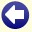## Solution to: Melting Snowballs

Let r1(t) be the radius of the smallest ball (ball 1) at time t.

Let r2(t) de the radius of the largest ball (ball 2) at time t.

Let r0 = r1(0).

Then holds r2(0) = 2 × r0. The surface Ai(t) of ball i at time t is equal to

4 × pi × (ri(t))2

and the volume Vi(t) of ball i at time t is equal to

4/3 × pi × (ri(t))3.

Then holds:

d Vi(t) / dt = - k × Ai(t)

so

d [4/3 × pi × (ri(t))3] / dt = - k × [4 × pi × (ri(t))2]

for a certain melting factor k independent of i. This gives

4 × pi × (ri(t))2 × [d ri(t) / dt] = - k × 4 × pi × (ri(t))2

so

[d ri(t) / dt] = - k

and

ri(t) = ri(0) - k × t.

Suppose that at time th half of the volume of ball 2 has melted, then

4/3 × pi × (r2(th))3 = 0.5 × 4/3 × pi × (r2(0))3

so

(r2(th))3 = 0.5 × (r2(0))3

and

(2 × r0 - k × th)3 = 4 × (r0)3.

Then holds:

k × th = 2 × r0 - 4(1/3) × r0.

At that time th holds for the small ball (ball 1):

r1(th) = r0 - k × th

= r0 - (2 × r0 - 4(1/3) × r0)

= 4(1/3) × r0 - r0

= (4(1/3) - 1) × r0.

The volume of ball 1 is at that time:

V1(t) = 4/3 × pi × (r1(t))3

= 4/3 × pi × ((4(1/3) - 1) × r0)3

= (4/3 × pi × r03) × (4(1/3) - 1)3

= (4(1/3) - 1)3 × V1(0)

so the volume of ball 1 at that moment is only (4(1/3) - 1)3 × 100% of the original volume. This is approximately 20.27%.Back to the puzzle
This website uses cookies. By further use of this website, or by clicking on 'Continue', you give permission for the use of cookies. If you want more information, look at our cookie policy.